# Neural ODEs: breakdown of another deep learning breakthrough

Source: Deep Learning on Medium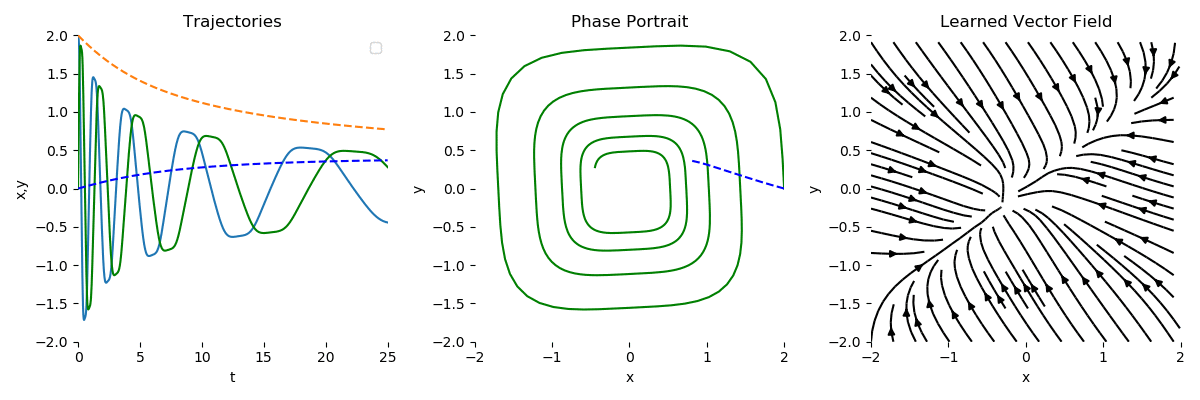Visualization of the Neural ODE learning the dynamical system

Hi everyone! If you’re reading this article, most probably you’re catching up with the recent advances that happen in the AI world. The topic we will review today comes from NIPS 2018, and it will be about the best paper award from there: Neural Ordinary Differential Equations (Neural ODEs). In this article, I will try to give a brief intro and the importance of this paper, but I will emphasize the practical use and how and for what we can apply this need breed of neural networks in applications and if can at all. As always, if you want to dive straight to the code, you can check this GitHub repository, I recommend you to launch it in Google Colab.

### Why do we care about ODEs?

First of all, let’s recap quickly what a beast ordinary differential equation is. It describes evolution in time of some process that depends on one variable (that’s why ordinary), and this change in time is described via a derivative:

Usually, we can talk about solving this differential equation, if we have some initial condition (at which point the process starts) and we want to see how the process will evolve up to some final state. The solution function is also called the integral curve (because we can integrate the equation to get the solution x(t)). Let’s try to solve the equation from the picture above using SymPy package:

`from sympy import dsolve, Eq, symbols, Function`
`t = symbols('t')x = symbols('x', cls=Function)deqn1 = Eq(x(t).diff(t), 1 - x(t))sol1 = dsolve(deqn1, x(t))`

which will return as the solution

`Eq(x(t), C1*exp(-t) + 1)`

where C1 is a constant, that can be determined while given some initial condition. ODEs can be solved analytically if given in the appropriate form, but normally they are solved numerically. One of the oldest and simplest algorithms is Euler’s method: the core idea is approximating the solution function step by step using tangents lines:

Visit the link under the picture for a more detailed explanation, but at the end, we end up with a very simple formula, for equation

the solution at the discretized grid of n time steps is

For more details on ODEs, especially how to program them and their solutions in Python, I recommend you to check out this book, it also has a lot of examples of processes in chemistry, physics and industrial fields that have such time evolution, that can be described with the ODEs. Also, for additional intuition about differential equation compared to ML models visit this resource. Meanwhile, looking at Euler’s equation doesn’t it remind you anything from the recent deep learning architectures yet…?

### ResNets are ODEs solutions?

Exactly! The y_{n+1} = y_n + f(t_n, y_n) is nothing but a residual connection in ResNet, where the output of some layer is a sum of the output of the layer f() itself and the input y_n to this layer. This is basically the main idea of neural ODEs: a chain of residual blocks in a neural network is basically a solution of the ODE with the Euler method! In this case, the initial condition for the system is “time” 0, which indicates the very first layer of the neural network, and as x(0) will serve the normal input, which can be time series, image, whatever you want! The final condition at “time” t will be the desired output of the neural network: a scalar value, a vector representing classes or anything else.

If we remember, that these residual connections are discretized time steps of the Euler method, it means, that we can regulate the depth of the neural network, just with choosing the discretizing scheme, hence, making the solution (aka neural network) more or less accurate, even making it infinite-layer like!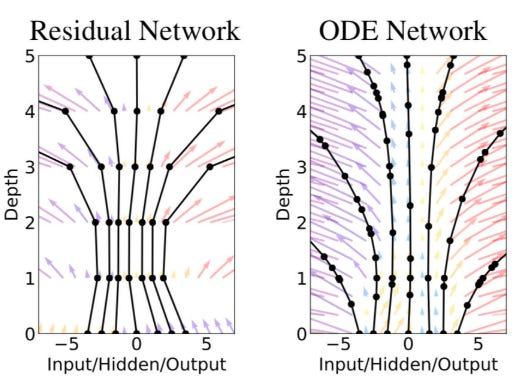Difference between ResNet with a fixed number of layers and ODENet with a flexible number of layer

Is Euler too primitive ODE solving method? Indeed it is, so let’s replace ResNet / EulerSolverNet with some abstract concept as ODESolveNet, where ODESolve will be a function, that provides a solution to the ODE (lowkey: our neural network itself) with much better accuracy than Euler’s method. The network architecture now might look like the following:

`nn = Network( Dense(...), # making some primary embedding ODESolve(...), # "infinite-layer neural network" Dense(...) # output layer)`

We forgot about one thing… A neural network is a differentiable function, so we can train it with gradient-based optimization routines. How should we backpropagate through the ODESolve() function, which is also actually a black box in our case? In particular, we need a gradient of loss function by the input and the dynamics parameters. The mathematical trick is called adjoint sensitivity method. I will refer you to the original paper and this tutorial for more details, but the essence is described in the picture below (L stands for the main loss function we want to optimize):

Briefly, alongside with the original dynamical system that describes the process, the adjoint system describes the derivative states at each point of the process backward, via the chain rule (that’s where the roots of well-known backpropagation are). Exactly from it, we can obtain the derivative by the initial state, and, in a similar way, by the parameters of a function that is modeling dynamics (one “residual block”, or the discretization step in the “old” Euler’s method).

For more details I recommend you to watch the presentation of one of the authors of the paper himself as well:

### Possible applications of neural ODEs

First, advantages and motivation to use them instead of the “normal ResNets”:

• Memory efficiency: we don’t need to store all the parameters and gradients while backpropagating
• Adaptive computation: we can balance speed and accuracy with the discretization scheme, moreover, having it different while training and inference
• Parameters efficiency: the parameters of nearby “layers” are automatically tied together (see the paper)
• Normalizing flows new type of invertible density models
• Continuous time series models: continuously-defined dynamics can naturally incorporate data which arrives at arbitrary times.

The applications, according to the paper, apart of the replacing ResNet with ODENet for computer vision, that I see a bit unrealistic now, are the next ones:

• Compressing complex ODEs into a single dynamic modeling neural network
• Applying it for time series with missing time steps
• Invertible normalizing flows (out of the scope of this blog)

For the disadvantages, consult the original paper, there are some. Enough theory, let’s check some practical examples now. Just to remind, all the code for experiments is here.

### Learning dynamical systems

As we could see before, differential equations are used widely do describe complex continuous processes. Of course, in real life we observe them as discrete processes, and, most importantly, a lot of observations at the time steps t_i can simply be missing. Let’s supposed you want to model such a system with a neural network. How would you deal with this kind of situation in a classical sequence modeling paradigm? Throw it onto the recurrent neural network somehow, which isn’t even designed for it. In this part, we will check how Neural ODEs will deal with them.

Our setup will be the following:

1. Define the ODE itself we will model as a PyTorch nn.Module()
2. Define a simple (or not really) neural network, that will model dynamics between two consequent dynamics steps from h_t to h_{t+1} or, in case of a dynamical system, x_t, and x_{t+1}.
3. Run the optimization process that backpropagates through the ODE solver and minimizes the difference between actual and modeled dynamics.

In all the following experiments the neural network will be just a following (which is supposedly enough to model simple functions with two variables):

`self.net = nn.Sequential( nn.Linear(2, 50), nn.Tanh(), nn.Linear(50, 2), )`

All further examples are highly inspired by this repository with amazing explanations. In the next subsections, I will show how the dynamical systems we model look themselves in the code and how system evolution over time and the phase portrait is being fit by the ODENet.

#### Simple spiral function

In this, and all the future visualizations, the dotted lines stand for the fitting model.

`true_A = torch.tensor([[-0.1, 2.0], [-2.0, -0.1]])`
`class Lambda(nn.Module): def forward(self, t, y): return torch.mm(y, true_A)`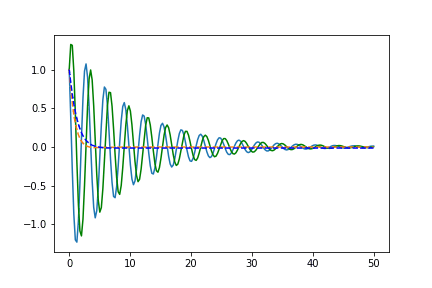Phase space on the left, time-space on the right. The straight line stands for the real trajectory and dotted one — for the evolution of the learned by the Neural ODE system

#### Random matrix function

`true_A = torch.randn(2, 2)/2.`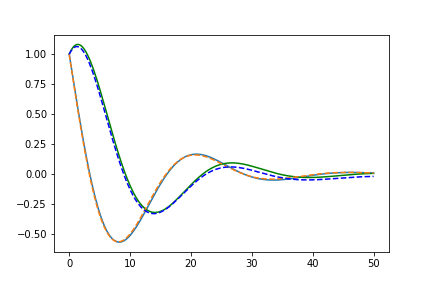Phase space on the left, time-space on the right. The straight line stands for the real trajectory and dotted one — for the evolution of the learned by the Neural ODE system

#### Volterra-Lotka system

`a, b, c, d = 1.5, 1.0, 3.0, 1.0true_A = torch.tensor([[0., -b*c/d], [d*a/b, 0.]])`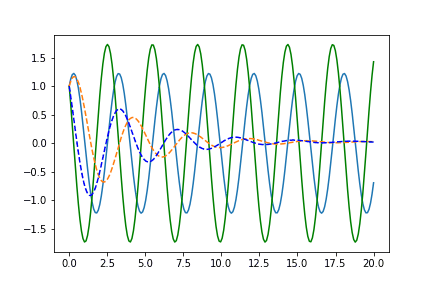Phase space on the left, time-space on the right. The straight line stands for the real trajectory and dotted one — for the evolution of the learned by the Neural ODE system

#### Nonlinear function

`true_A2 = torch.tensor([[-0.1, -0.5], [0.5, -0.1]])true_B2 = torch.tensor([[0.2, 1.], [-1, 0.2]])`
`class Lambda2(nn.Module): def __init__(self, A, B): super(Lambda2, self).__init__() self.A = nn.Linear(2, 2, bias=False) self.A.weight = nn.Parameter(A) self.B = nn.Linear(2, 2, bias=False) self.B.weight = nn.Parameter(B) def forward(self, t, y): xTx0 = torch.sum(y * true_y0, dim=1) dxdt = torch.sigmoid(xTx0) * self.A(y - true_y0) + torch.sigmoid(-xTx0) * self.B(y + true_y0) return dxdt`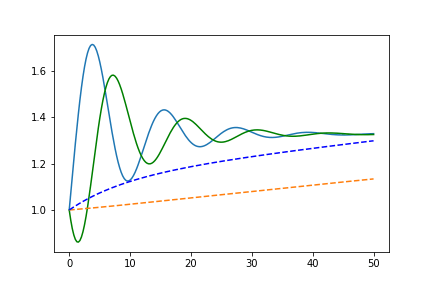Phase space on the left, time-space on the right. The straight line stands for the real trajectory and dotted one — for the evolution of the learned by the Neural ODE system

As we can see, our single “residual block” can’t learn this process well enough, so we might make it more complex for the next functions.

#### Neural network function

Let’s make a function fully parametrized by a multilayer perceptron with randomly initialized weights:

`true_y0 = torch.tensor([[1., 1.]])t = torch.linspace(-15., 15., data_size)`
`class Lambda3(nn.Module): def __init__(self): super(Lambda3, self).__init__() self.fc1 = nn.Linear(2, 25, bias = False) self.fc2 = nn.Linear(25, 50, bias = False) self.fc3 = nn.Linear(50, 10, bias = False) self.fc4 = nn.Linear(10, 2, bias = False) self.relu = nn.ELU(inplace=True) def forward(self, t, y): x = self.relu(self.fc1(y * t)) x = self.relu(self.fc2(x)) x = self.relu(self.fc3(x)) x = self.relu(self.fc4(x)) return x`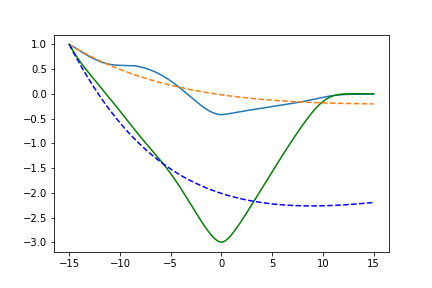Phase space on the left, time-space on the right. The straight line stands for the real trajectory and dotted one — for the evolution of the learned by the Neural ODE system

Here 2–50–2 network fails horribly because it’s too simple, let’s increase its depth:

`self.net = nn.Sequential( nn.Linear(2, 150), nn.Tanh(), nn.Linear(150, 50), nn.Tanh(), nn.Linear(50, 50), nn.Tanh(), nn.Linear(50, 2), )`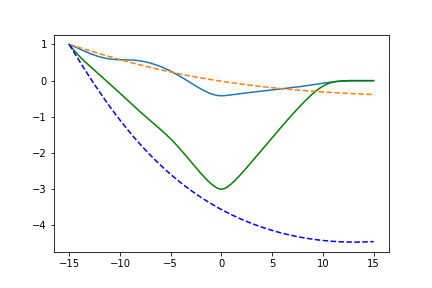Phase space on the left, time-space on the right. The straight line stands for the real trajectory and dotted one — for the evolution of the learned by the Neural ODE system

Now it works more or less as expected, don’t forget to check the code :)

### Neural ODEs as generative models

Authors also claim that they can build a generative time series model via VAE framework, using Neural ODEs as a part of it. How does it work?

• First, we encode the input sequence with some “standard” time series algorithms, let’s say RNN to obtain the primary embedding of the process
• Run the embedding through the Neural ODE to get the “continuous” embedding
• Recover initial sequence from the “continuous” embedding in VAE fashion

As a proof of concept, I just have re-run the code from this repository and it seemed like working very well in learning spiral trajectories: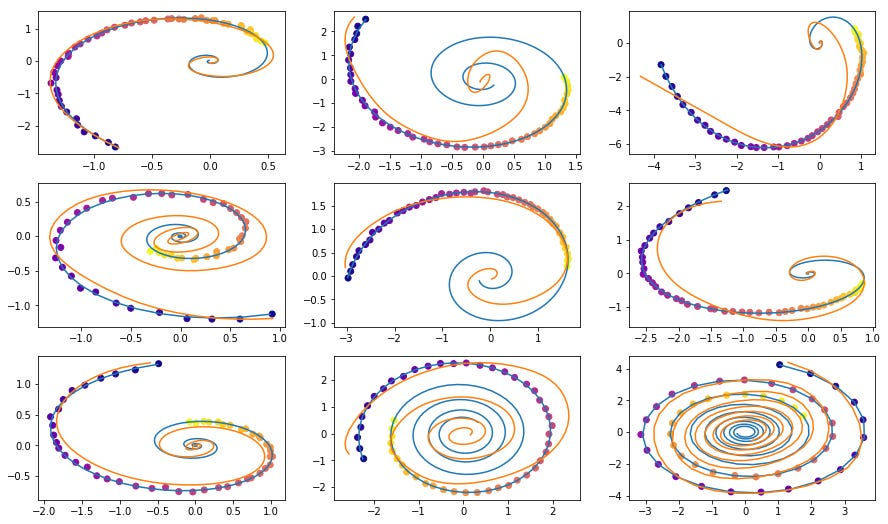Dots are sampled noisy trajectories, Blue line is true trajectory, the orange line stands for recovered and interpolated trajectory

Then, I decided to turn heartbeats from the electrocardiogram (ECG) to the phase portraits with x(t) as time-space and x`(t) as derivative-space (as it was presented in this work) and tried to fit with different VAE setting. This use case might be very useful for wearable devices like Mawi Band, where due to noisy or interrupted signal we have to recover it (and actually we do it with the help of deep learning, but ECG is a continuous signal, isn’t it?). Unfortunately, it doesn’t converge really well, showing all the signs of overfitting to a single form of a beat: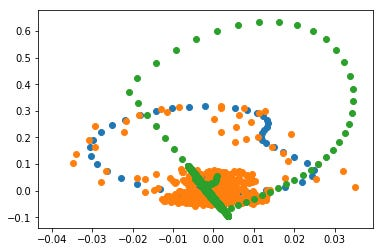Phase spaces. Blue line — real trajectory, orange line — sampled and noisy trajectory, green line — auto-encoded trajectory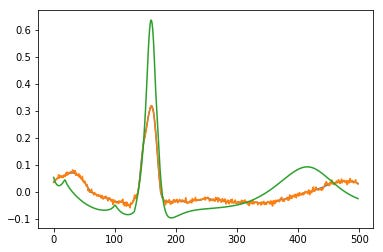Time spaces. Blue line — real signal, orange line — sampled and noisy signal, green line — auto-encoded signal

I also have tried another experiment: to learn this autoencoder only on the parts of each beat and recover the whole waveform from it (i.e. let’s extrapolate a signal). Unfortunately, I didn’t come up with anything meaningful with extrapolating this piece of signal to the left or to the right — just collapsing to infinity, whatever I did with the hyperparameters and data preprocessing. Probably, someone of the readers may help to understand what went wrong :(

### What’s next?

It’s clear, that Neural ODEs are designed to learn relatively simple processes (that’s why we even have ordinary in the title), so we need a model that is able to model much richer families of functions. And there are two interesting approaches already:

Will take some time to explore them as well :)

### Conclusions

IMHO, Neural ODEs are not ready to be used in practice yet. The idea itself is great and by the level of innovation it reminds me of the Capsule Networks by Geoffrey Hinton, but where they are now…? As well as Neural ODEs they showed good results on the toy tasks but failed on anything close to real applications or large-scale datasets.

I can see only two practical application at the moment:

• using the ODESolve() layers to balance speed/accuracy tradeoff in classical neural networks
• “squeezing” regular ODEs into the neural architectures to embed them in standard data science pipelines

Personally, I am hoping to the further development of this direction (I showed some links above) to make these Neural (O)DEs represent much richer classes of functions and I will follow it closely.

P.S.
Follow me also on the Facebook blog, where I regularly post short AI articles or news that are too short for Medium, Instagram for personal stuff and Linkedin! Contact me if you want to collaborate on interpretable AI applications or other ML projects.